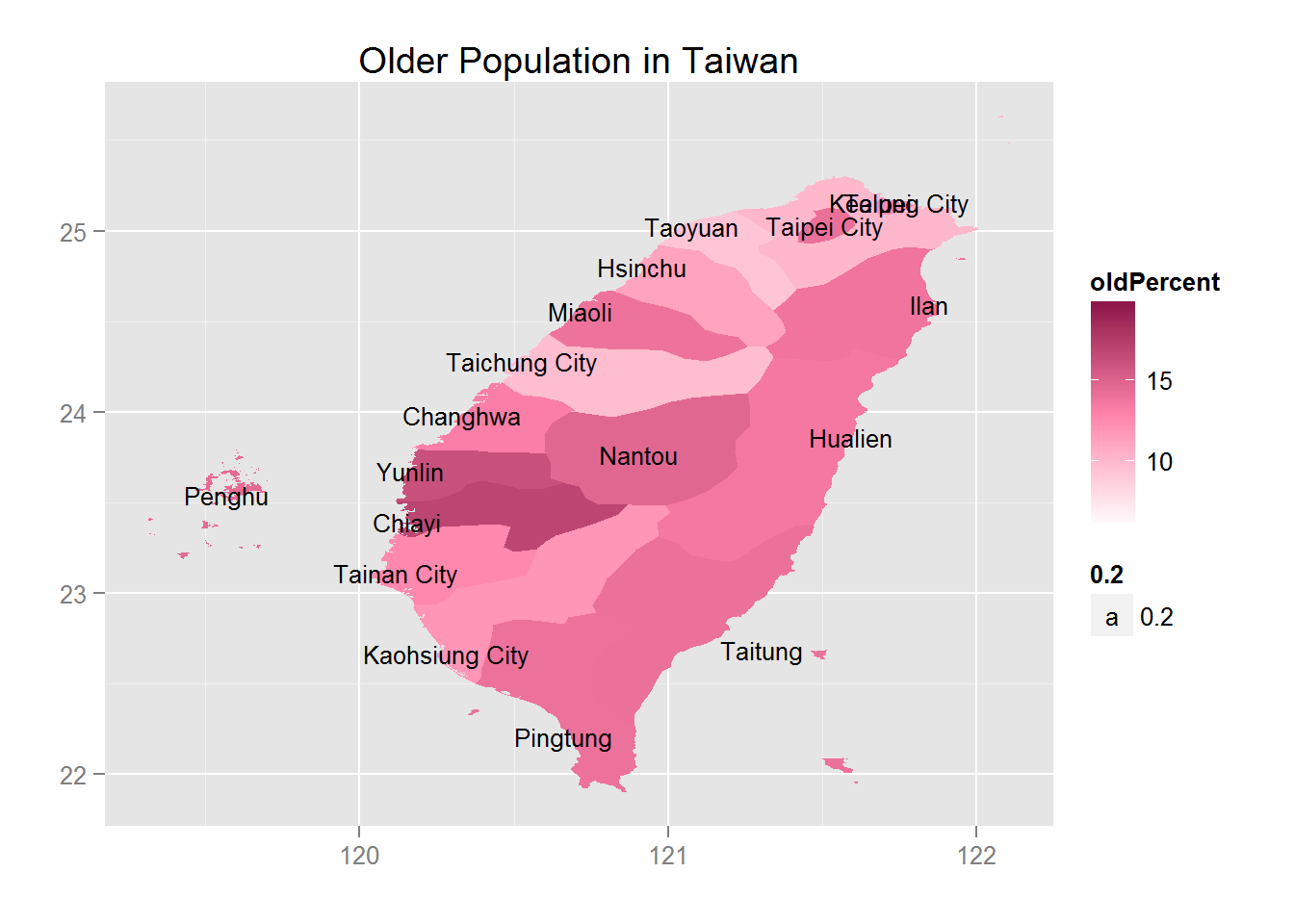# Introduction

There are plenty of ways to make choropleth maps in R. This example demonstrates the easiest way for beginners in my point of view. In this article, we will first load required packages, introduce GADM.org and its resources, then use a census of National Statistics, Taiwan to map a simple choropleth map to indicate the percentage of older population(say, over 65 years old) in each administrative area.

# Required Packages

packageNames <- c("plyr", "ggplot2","rgeos", "maptools", "scales", "raster")
lapply(packageNames, library, character.only=TRUE)

GADM is a spatial database of the location of the world’s administrative areas (or adminstrative boundaries) for use in GIS and similar software. Administrative areas in this database are countries and lower level subdivisions.

## Get Data from GADM with raster

You can find that there are three levels of SpatialPolygonDataFrame for Taiwan here. Let us get all three of them and see what are the differences between them.

twDist0 <- getData('GADM', country='TW', level=0)
twDist2 <- getData('GADM', country='TW', level=2)

Let us plot all of them.

plot(twDist0)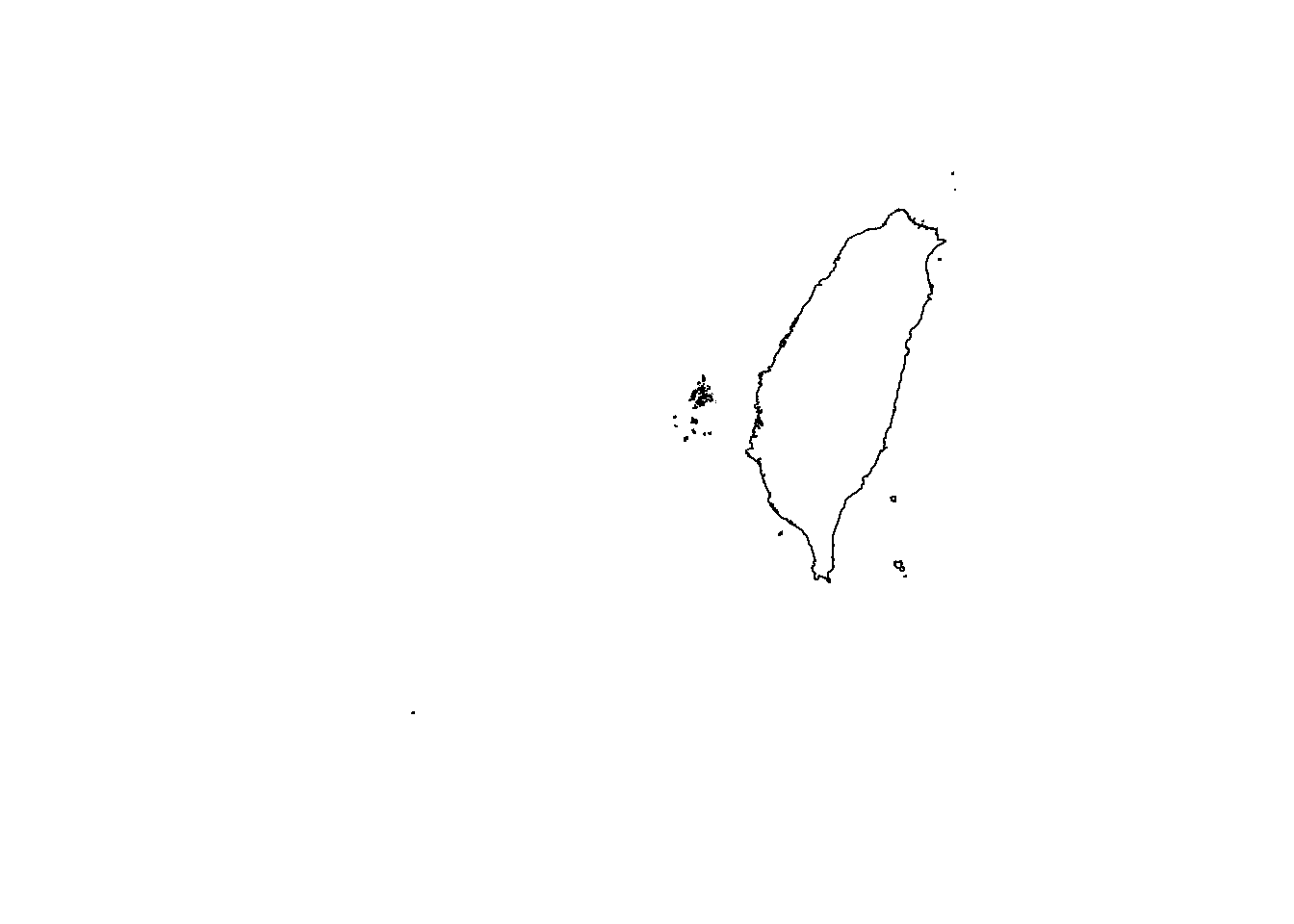plot(twDist1)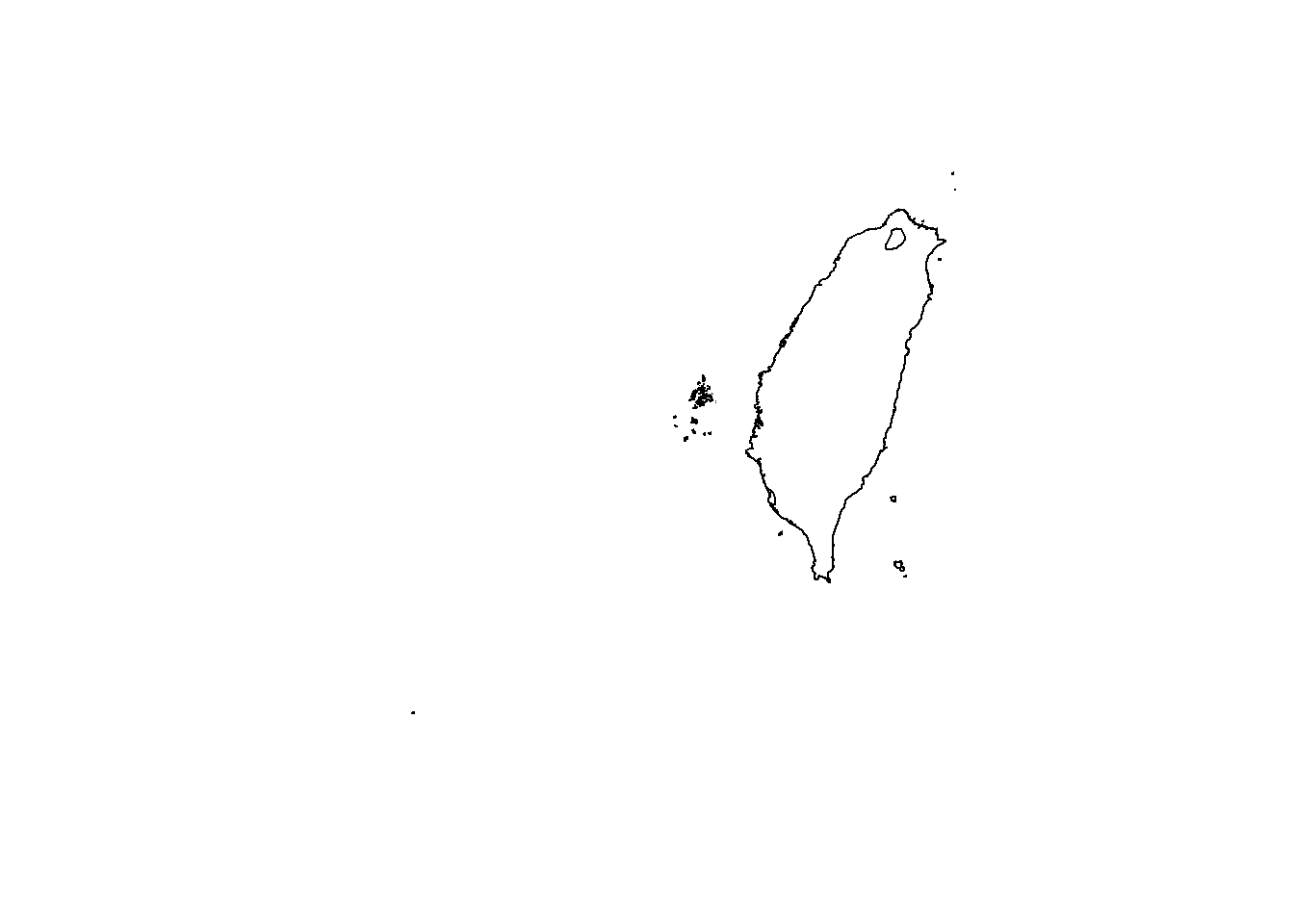plot(twDist2)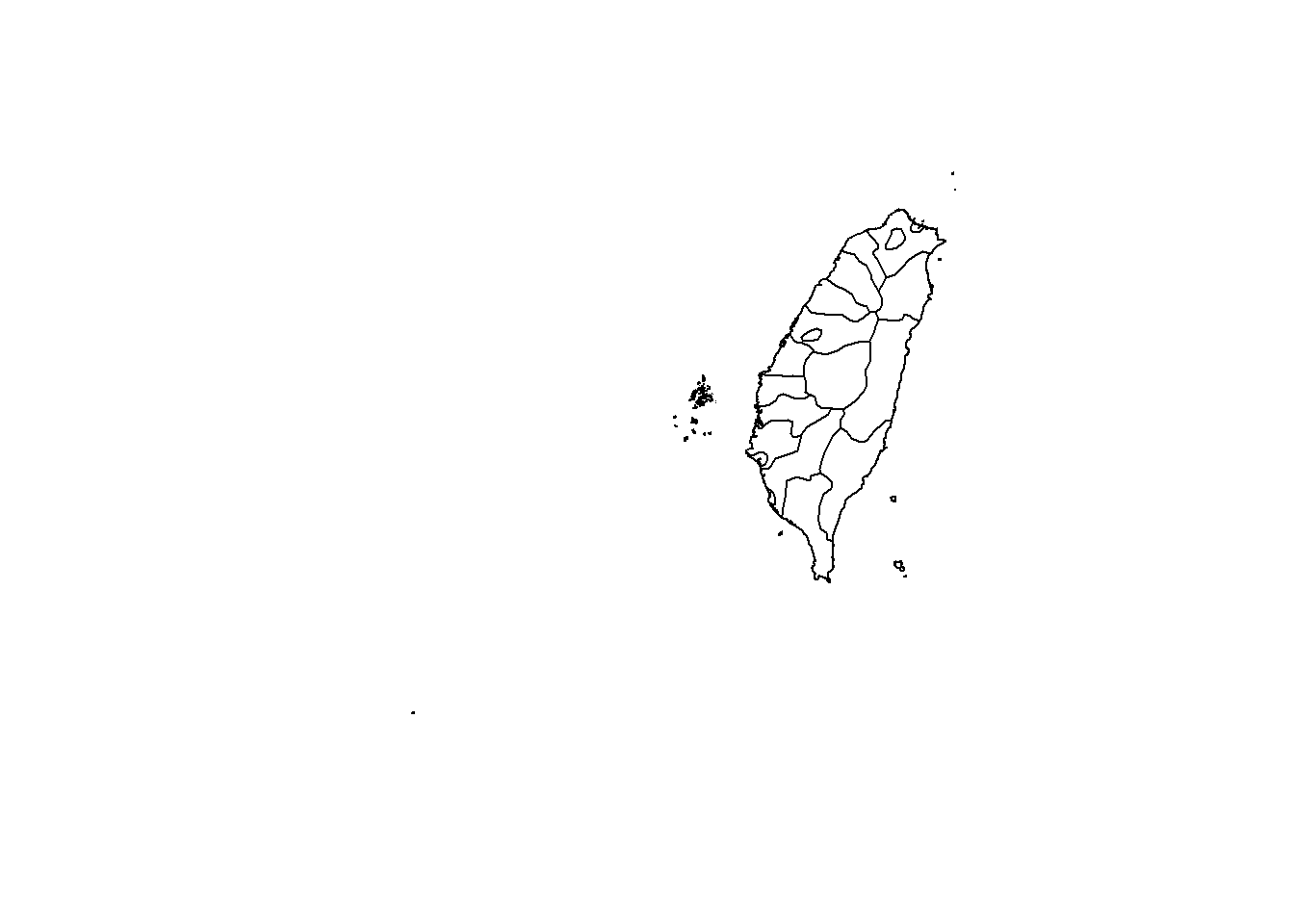Now you can see the difference clearly and we will use the level2 data for our choropleth map.

# Data of National Statistics, Taiwan

We downloaded our data from National Statistics, Taiwan. After a bit of manipulation, the data looks like this.

setwd("C:/Users/ASUS/Documents/ApplicationInR/countyIndicatorTW")
head(twIndex)
##               id oldPercent socialIncrease unemployment
## 1         Taipei      10.10          -1.21          3.9
## 2    Taipei City      14.08           1.47          4.0
## 3        Taoyuan       9.31           4.29          4.0
## 4  Taichung City       9.79           2.93          3.9
## 5    Tainan City      12.62           0.02          4.1
## 6 Kaohsiung City      11.95          -1.12          3.9

We will use the variable oldPercent.

# Data Manipulation

The administrative area of Taiwan somehow is a bit different to the one downloaded from GADM.org. So we will modify our map data and statistics data accordingly.

twDist2 <- fortify(twDist2, region = "NAME_2")#fortify function helps us transform a SpatialPolygonDataFrame into a data frame, which is easier to manipulate.
twDist2$id[twDist2$id=='Taichung'] <- 'Taichung City'
twDist2$id[twDist2$id=='Tainan'] <- 'Tainan City'
twDist2$id[twDist2$id=='Kaohsiung'] <- 'Kaohsiung City'
twIndexAvg <- ddply(twIndex, .(id), summarize, oldPercentAvg = mean(oldPercent), socialIncreaseAvg=mean(socialIncrease), unemploymentAvg=mean(unemployment))#We just roughly average the indicators of 2 counties if they are merged in data from GADM.

# Plot with ggplot2

ggplot() + geom_map(data=twIndex, aes(map_id = id, fill = oldPercent), map = twDist2) + expand_limits(x = twDist2$long, y = twDist2$lat)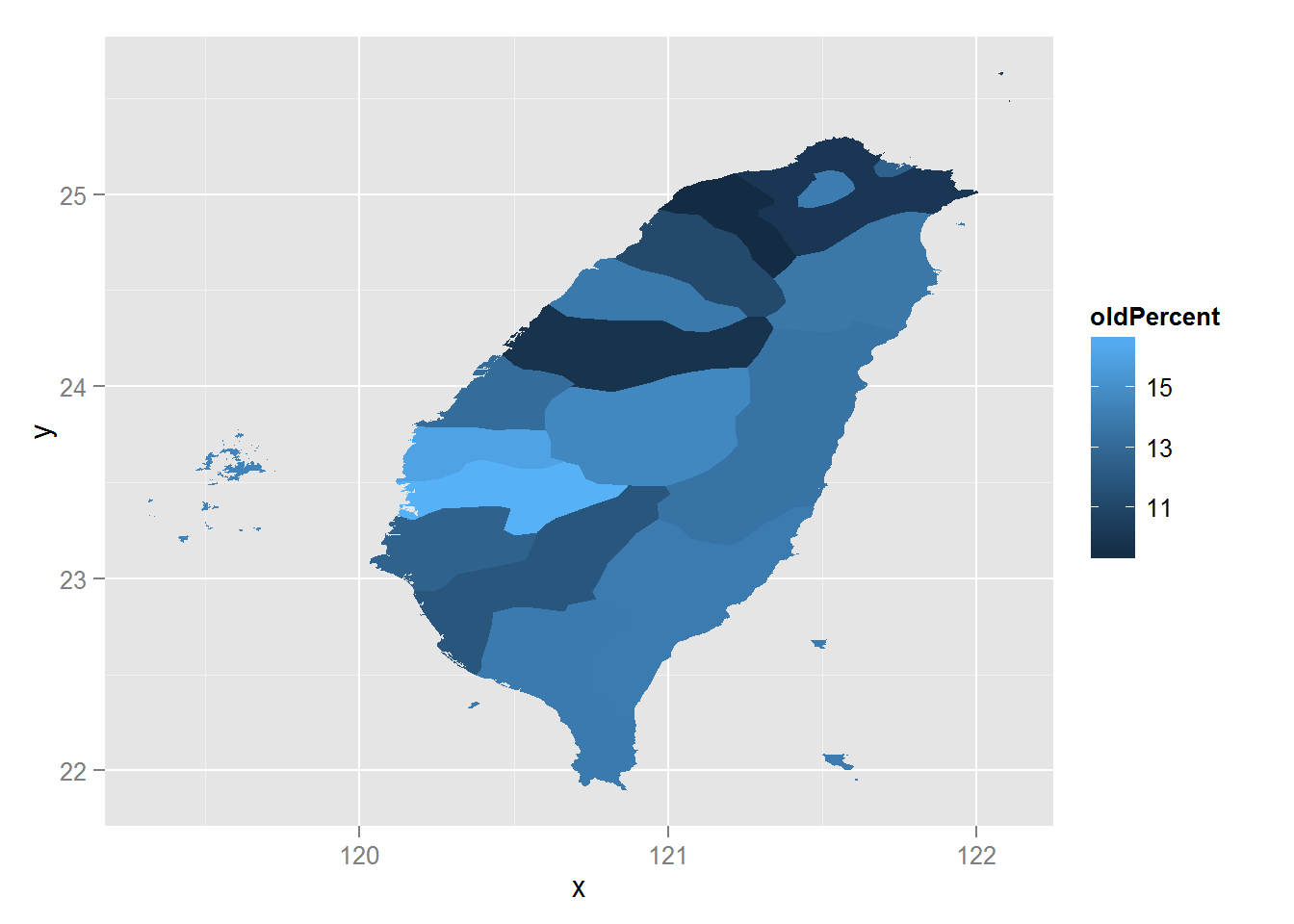## Make it Prettier

Let’s make the choropleth map prettier by revising its color and putting tags of county on it.

distanceCenter <- ddply(twDist2, .(id), summarize, latCenter = mean(lat), longCenter = mean(long))
ggplot() + geom_map(data=twIndex, aes(map_id = id, fill = oldPercent), map = twDist2) + expand_limits(x = twDist2$long, y = twDist2$lat) + scale_fill_gradient2(low = "white",  mid = "palevioletred1", midpoint = mean(twIndex$oldPercent), high = muted("palevioletred4"), limits = c(min(twIndex$oldPercent)-3, max(twIndex\$oldPercent)+3))+geom_text(data = distanceCenter, aes(x = longCenter, y = latCenter, label = id, size = 0.2))+xlab("")+ylab("")+ggtitle("Older Population in Taiwan")